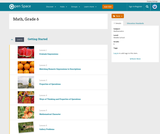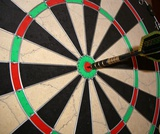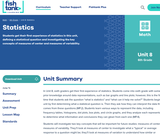# 8 Results

View
Selected filters:
• Box PlotConditional Remix & Share Permitted
CC BY-NC
Rating

Subject:
Mathematics
Provider:
Pearson
02/28/2022Rating

Distributions and Variability

Type of Unit: Project

Prior Knowledge

Students should be able to:

Represent and interpret data using a line plot.
Understand other visual representations of data.

Lesson Flow

Students begin the unit by discussing what constitutes a statistical question. In order to answer statistical questions, data must be gathered in a consistent and accurate manner and then analyzed using appropriate tools.

Students learn different tools for analyzing data, including:

Measures of center: mean (average), median, mode
Measures of spread: mean absolute deviation, lower and upper extremes, lower and upper quartile, interquartile range
Visual representations: line plot, box plot, histogram

These tools are compared and contrasted to better understand the benefits and limitations of each. Analyzing different data sets using these tools will develop an understanding for which ones are the most appropriate to interpret the given data.

To demonstrate their understanding of the concepts, students will work on a project for the duration of the unit. The project will involve identifying an appropriate statistical question, collecting data, analyzing data, and presenting the results. It will serve as the final assessment.

Subject:
Mathematics
Statistics and Probability
Provider:
PearsonConditional Remix & Share Permitted
CC BY-NC
Rating

Students make a box plot for their typical-sixth-grader data from Lesson 7 and write a summary of what the plot shows.Using the line plot from Lesson 4, students construct a box plot. Students learn how to calculate the five-number summary and interquartile range (IQR). Students apply this knowledge to the data used in Lesson 7 and describe the data in terms of the box plot. Class discussion focuses on comparing the two graphs and what they show for the sets of data.Key ConceptsA box-and-whisker plot, or box plot, shows the spread of a set of data. It shows five key measures, called the five-number summary.Lower extreme: The smallest value in the data setLower quartile: The middle of the lower half of the data, and the value that 25% of the data fall belowMedian: The middle of the data setUpper quartile: The middle of the upper half of the data, and the value that 25% of the data are aboveUpper extreme: The greatest value in the data setThis diagram shows how these values relate to the parts of a box plot.The length of the box represents the interquartile range (IQR), which is the difference between the lower and upper quartile.A box plot divides the data into four equal parts. One quarter of the data is represented by the left whisker, two quarters by each half of the box, and one quarter by the right whisker. If one of these parts is long, the data in that quarter are spread out. If one of these quarters is short, the data in that quarter are clustered together.Goals and Learning ObjectivesLearn how to construct box plots, another tool to describe data.Learn about the five-number summary, interquartile range, and how they are related to box plots.Compare a line plot and box plot for the same set of data.

Subject:
Statistics and Probability
Material Type:
Lesson Plan
Provider:
Pearson
Author:
02/28/2022Conditional Remix & Share Permitted
CC BY-NC
Rating

Groups begin presentations for their unit project. Students provide constructive feedback on others' presentations.Key ConceptsThe unit project serves as the final assessment. Students should demonstrate their understanding of unit concepts:Measures of center (mean, median, mode) and spread (MAD, range, interquartile range)The five-number summary and its relationship to box plotsRelationship between data sets and line plots, box plots, and histogramsAdvantages and disadvantages of portraying data in line plots, box plots, and histogramsGoals and Learning ObjectivesPresent projects and demonstrate an understanding of the unit concepts.Provide feedback for others' presentations.Review the concepts from the unit.

Subject:
Statistics and Probability
Material Type:
Lesson Plan
Provider:
Pearson
Author:
02/28/2022Conditional Remix & Share Permitted
CC BY-NC
Rating

Remaining groups present their unit projects. Students discuss teacher and peer feedback.Key ConceptsThe unit project serves as the final assessment. Students should demonstrate their understanding of unit concepts:Measures of center (mean, median, mode) and spread (MAD, range, interquartile range)The five-number summary and its relationship to box plotsRelationship between data sets and line plots, box plots, and histogramsAdvantages and disadvantages of portraying data in line plots, box plots, and histogramsGoals and Learning ObjectivesPresent projects and demonstrate an understanding of the unit concepts.Provide feedback for others' presentations.Review the concepts from the unit.Review presentation feedback and reflect.

Subject:
Statistics and Probability
Material Type:
Lesson Plan
Provider:
Pearson
Author:
02/28/2022Conditional Remix & Share Permitted
CC BY-NC
Rating

Students use the Box Plot interactive, which allows them to create line plots and see the corresponding box plots. They use this tool to create data sets with box plots that satisfy given criteria.Students investigate how the box plot changes as the data points in the line plot are moved. Students can manipulate data points to change aspects of the box plot and to see how the line plot changes. Students create box plots that fit certain criteria.Key ConceptsThis lesson focuses on the connection between a data set and its box plot. It reinforces the idea that a box plot shows the spread of a data set, but not the individual data points.Students will observe the following similarities and differences between line plots and box plots:Line plots allow us to see and count individual values, while box plots do not.Line plots allow us to find the mean and the mode of a set of data, while box plots do not.Box plots are useful for very large data sets, while line plots are not.Box plots give us a better picture of how the values in a data set are distributed than line plots do, and they allow us to see measures of spread easily.Goals and Learning ObjectivesExperiment with different line plots to see the effect on the corresponding box plots.Create data sets with box plots that satisfy different criteria.Compare and contrast line plots and box plots.

Subject:
Statistics and Probability
Material Type:
Lesson Plan
Provider:
Pearson
Author:
02/28/2022Conditional Remix & Share Permitted
CC BY-NC
Rating

Students analyze the data they have collected to answer their question for the unit project. They will also complete a short Self Check.Students are given class time to work on their projects. Students should use the time to analyze their data, finding the different measures and/or graphing their data. If necessary, students may choose to use the time to collect data. Students also complete a short pre-assessment (Self Check problem).Key ConceptsStudents will look at all of the tools that they have to analyze data. These include:Graphic representations: line plots, box plots, and histogramsMeasures of center and spread: mean, median, mode, range, and the five-number summaryStudents will use these tools to work on their project and to complete an assessment exercise.Goals and Learning ObjectivesComplete the project, or progress far enough to complete it outside of class.Review measures of center and spread and the three types of graphs explored in the unit.Check  knowledge of box plots and measures of center and spread.

Subject:
Statistics and Probability
Material Type:
Lesson Plan
Provider:
Pearson
Author:
02/28/2022Conditional Remix & Share Permitted
CC BY-NC-SA
Rating

Students get their first experience of statistics in this unit, defining a statistical question and investigating the key concepts of measures of center and measures of variability.

Subject:
Mathematics
Material Type:
Unit of Study
Provider:
Fishtank Learning
Provider Set:
Mathematics